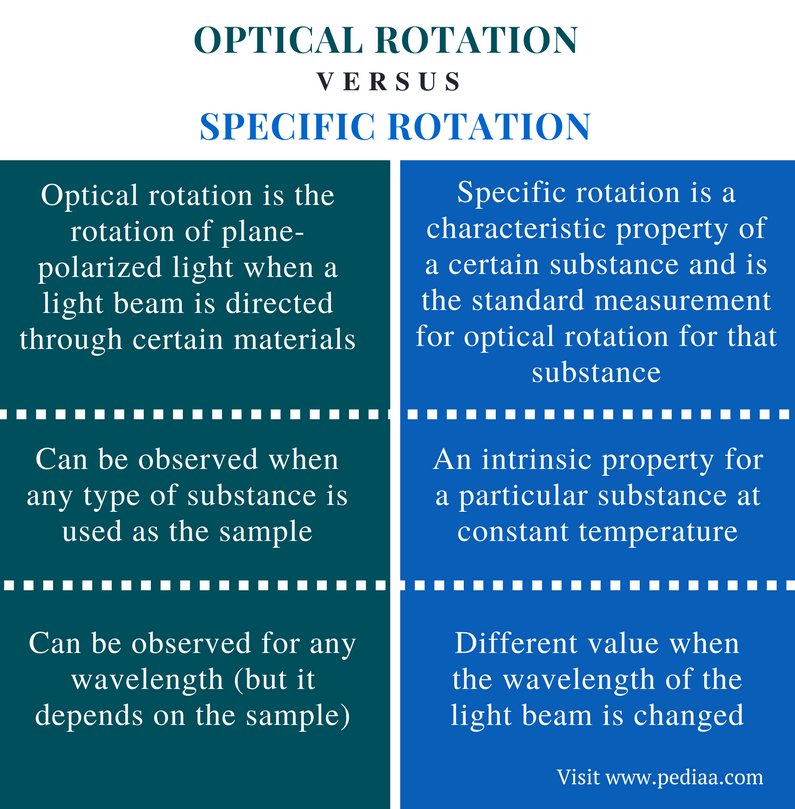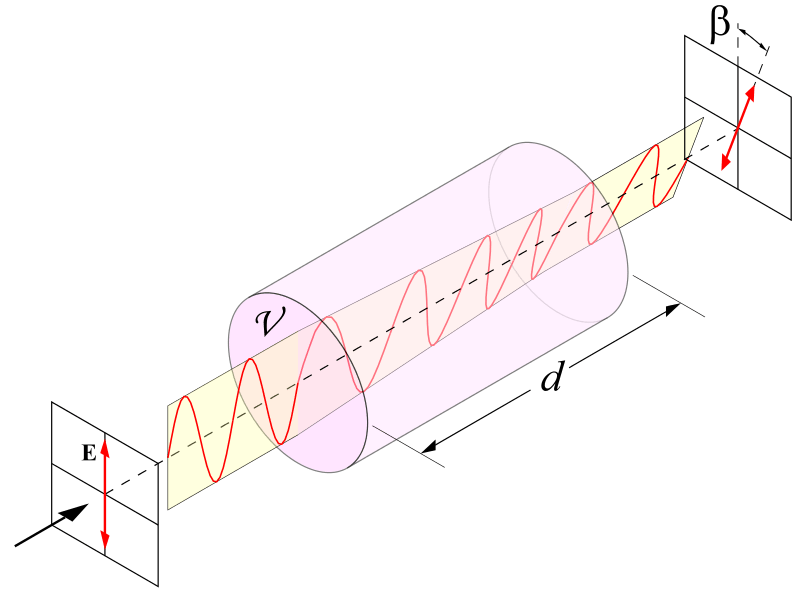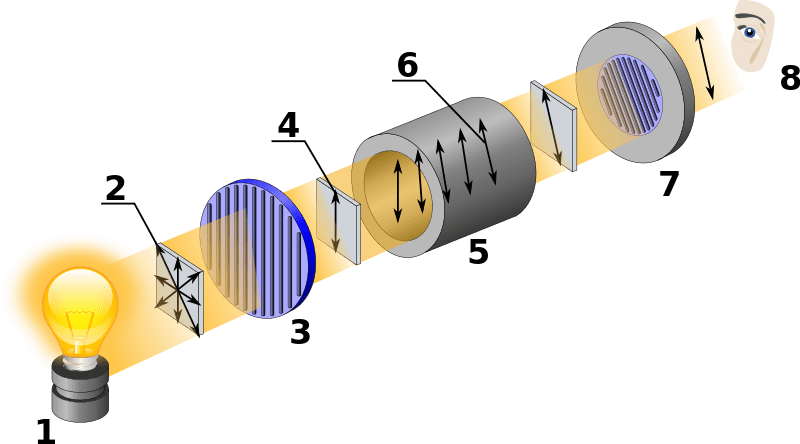# Difference Between Optical Rotation and Specific Rotation

## Main Difference – Optical Rotation vs Specific Rotation

Both optical rotation and specific rotation express the same idea of rotating the plane-polarized light into different directions by certain substances. These substances are called optical isomers or enantiomers. Optical rotation is the rotation of plane-polarized light when a light beam is directed through certain materials. Specific rotation gives the angle of rotation of plane-polarized light by a certain compound at a certain temperature. This is the key difference between optical rotation and specific rotation. The standard measurement for optical rotation for a specific chemical compound is called the specific rotation.

### Key Areas Covered

1. What is Optical Rotation
– Definition, Explanation
2. What is Specific Rotation
– Definition, Explanation, and Calculation
3. What is the Difference Between Optical Rotation and Specific Rotation
– Comparison of Key Differences

Key Terms: Dextrorotary, Enantiomers, Intrinsic Property, Levorotary, Optical Isomers, Optical Rotation, Plane-Polarized Light, Polarimeter, Specific Rotation## What is Optical Rotation

Optical rotation is also known as optical activity. It is the rotation of plane-polarized light when a light beam is directed through certain materials. These materials are known as optically active compounds. The compounds that can be included in this group are called enantiomers or optical isomers.

Enantiomer or optical isomers have the same arrangement of atoms but their 3D molecular structures are different from each other. Therefore, a pair of enantiomers is non-superimposable mirror images of each other. These isomers have the same chemical and physical properties except for their optical activity.Figure 1: Optical Rotation

When a pair of enantiomers is considered, one compound rotates plane polarized light in a clockwise direction whereas the other enantiomer rotates plane polarized light in an anticlockwise direction. Depending on the direction to which the plane-polarized light is rotated, the enantiomers are named. The compound that can rotate light in the clockwise direction is named as either the (+) isomer or the d-isomer. The letter d is used to express the word “dextrorotary” (clockwise). The compound that can rotate light in the anticlockwise direction is named as either the (-) isomer or the l-isomer. The letter l is used to express the word “levorotary” (anticlockwise).

The optical rotation is measured by polarimetry using a polarimeter. Here, a plane-polarized light beam is directed through a sample and the rotation of that light beam is measured. The final measurement depends on several factors such as the concentration of the sample, wavelength of the light that is used, temperature, etc.

## What is Specific Rotation

Specific rotation is a characteristic property of a certain substance and it is the standard measurement for optical rotation for that substance. Specific rotation gives the change in the direction of plane-polarized light per unit distance of cell and per unit concentration of the sample when light is passed through that sample. Specific rotation is an intrinsic property. This means it is a property that a substance has of itself, independently of other things.Figure 2: Mechanism of Optical Rotation in a Polarimeter

1 is a normal light source (unpolarized) and it is polarized by a prism polarizer (at 2). The sample is kept in a cell (at 5) where optical rotation occurs. Finally, it is detected by a prism analyzer (at 7).

### Specific Rotation Formula and Calculation

The specific rotation of a substance is given by the following formula.

[α]Tλ  =  α / l.c

Where, α is the observer rotation of plane-polarized light,

l is the path length of the light or the length of the sample cell,

c is the concentration or the density of the sample.

T is the temperature and λ is the wavelength of the light. In order to get the value for specific rotation of a substance, the temperature and the wavelength (of incident light) should be constant. The specific rotation is also measured using a polarimeter.

## Difference Between Optical Rotation and Specific Rotation

### Definition

Optical Rotation: Optical rotation is the rotation of plane-polarized light when a light beam is directed through certain materials.

Specific Rotation: Specific rotation is a characteristic property of a certain substance and it is the standard measurement for optical rotation for that substance.

### Nature

Optical Rotation: Optical rotation can be observed when any type of substance is used as the sample.

Specific Rotation:  Specific rotation is an intrinsic property for a particular substance at constant temperature.

### Wavelength

Optical Rotation:  The optical rotation can be observed for any wavelength (but it depends on the sample).

Specific Rotation:  The specific rotation is a different value when the wavelength of the light beam is changed.

### Conclusion

Optical rotation is the rotation of plane-polarized light by a substance. This rotation can be either clockwise or anticlockwise. The compounds that are capable of this rotation are enantiomers. The standard measurement for optical rotation of a specific chemical compound is called specific rotation.  This is the basic difference between optical rotation and specific rotation.

##### References:

1. Chirality and Optical Activity, chemed, Available here.
2. Libretexts. “Optical Activity.” Chemistry LibreTexts, Libretexts, 6 Aug. 2016, Available here.
3. “Specific Rotation: Formula & Calculation.” Study.com, Available here.

##### Image Courtesy:

1. “Optical-rotation” By User:DrBob~commonswiki (CC BY-SA 3.0) via Commons Wikimedia
2. “Polarimeter (Optical rotation)” By Kaidor – (CC BY-SA 3.0) via Commons Wikimedia